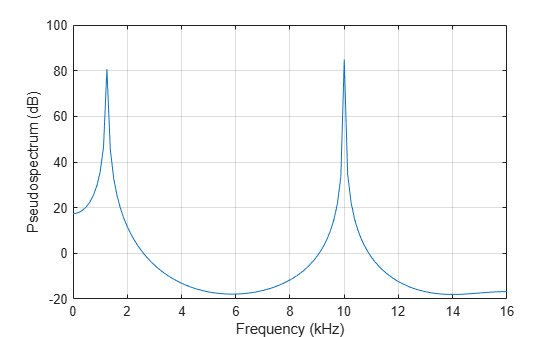# dspdata.pseudospectrum

Pseudospectrum `dspdata` object

## Syntax

```Hps = dspdata.pseudospectrum(Data) Hps = dspdata.pseudospectrum(Data,Frequencies) Hps = dspdata.pseudospectrum(...,'Fs',Fs) Hps = dspdata.pseudospectrum(...,'SpectrumRange',SpectrumRange) Hps = dspdata.pseudospectrum(...,'CenterDC',flag) ```

## Description

Note

The use of `dspdata.pseudospectrum` is not recommended. Use `peig` or `pmusic` instead.

A pseudospectrum is an indicator of the presence of sinusoidal components in a signal.

`Hps = dspdata.pseudospectrum(Data)` uses the pseudospectrum data contained in `Data`, which can be in the form of a vector or a matrix, where each column is a separate set of data. Default values for other properties of the object are:

Property

Default Value

Description

Name

`'Pseudospectrum'`

`Frequencies`

`[]`

type `double`

Vector of frequencies at which the power spectral density is evaluated. The range of this vector depends on the `SpectrumRange` value. For half, the default range is [0, π) or [0, `Fs`/2) for odd length, and [0, π] or [0, `Fs`/2] for even length, if `Fs` is specified. For whole, it is [0, 2π) or [0, `Fs`).

If you do not specify `Frequencies`, a default vector is created. If half the Nyquist range is selected, then the whole number of FFT points (nFFT) for this vector is assumed to be even.

If `half` the Nyquist range is selected and you specify `Frequencies`, the last frequency point is compared to the next-to-last point and to π (or `Fs`/2, if `Fs` is specified). If the last point is closer to π (or `Fs`/2) than it is to the previous point, nFFT is assumed to be even. If it is closer to the previous point, nFFT is assumed to be odd.

The length of the `Frequencies` vector must match the length of the columns of `Data`.

`Fs`

`'Normalized'`

Sampling frequency, which is `'Normalized'` if `NormalizedFrequency` is `true`. If `NormalizedFrequency` is `false` `Fs` defaults to `1`.

`SpectrumRange`

`'Half'`

Nyquist interval over which the pseudospectrum is calculated. Valid values are `'Half'` and `'Whole'`. See the `half` and `whole` methods in `dspdata` for information on changing this property.

The interval for `Half` is [0 π) or [0 π] depending on the number of FFT points, and for `Whole` the interval is [0 2π).

`NormalizedFrequency`

`true`

Whether the frequency is normalized (`true`) or not (`false`). This property is set automatically at construction time based on `Fs`. If `Fs` is specified, `NormalizedFrequency` is set to `false`. See the `normalizefreq` method in `dspdata` for information on changing this property.

`Hps = dspdata.pseudospectrum(Data,Frequencies)` uses the pseudospectrum estimation data contained in the `Data` and `Frequencies` vectors.

`Hps = dspdata.pseudospectrum(...,'Fs',Fs)` uses the sampling frequency `Fs`. Specifying `Fs` uses a default set of linear frequencies (in `Hz`) based on `Fs` and sets `NormalizedFrequency` to `false`.

`Hps = dspdata.pseudospectrum(...,'SpectrumRange',SpectrumRange)` uses the `SpectrumRange` argument to specify the interval over which the pseudospectrum was calculated. For data that ranges from [0 π) or [0 π], set the `SpectrumRange` to `half`; for data that ranges from [0 2π), set the `SpectrumRange` to `whole`.

`Hps = dspdata.pseudospectrum(...,'CenterDC',flag)` uses the value of `flag` to indicate whether the zero-frequency (DC) component is centered. If `flag` is `true`, it indicates that the DC component is in the center of the whole Nyquist range spectrum. Set the `flag` to `false` if the DC component is on the left edge of the spectrum.

### Methods

Methods provide ways of performing functions directly on your `dspdata` object. You can apply a method directly on the variable you assigned to your `dspdata.pseudospectrum` object. You can use the following methods with a `dspdata.pseudospectrum` object.

• `centerdc`

• `halfrange`

• `normalizefreq`

• `plot`

• `wholerange`

For example, to normalize the frequency and set the `NormalizedFrequency` parameter to true, use

```Hps = normalizefreq(Hps) ```

For detailed information on using the methods and plotting the pseudospectrum, see the `dspdata` reference page.

## Examples

collapse all

Use eigenanalysis to estimate the pseudospectrum of a noisy sinusoidal signal with two frequency components.

```Fs = 32e3; t = 0:1/Fs:2.96; x = cos(2*pi*t*1.24e3) + cos(2*pi*t*10e3) + randn(size(t)); P = pmusic(x,4);```

Create a `pseudospectrum` data object to store the results. Plot the pseudospectrum.

```hps = dspdata.pseudospectrum(P,'Fs',Fs); plot(hps)```## Version History

Introduced before R2006a